The compound of three octahedra and a remarkable compound of three square dipyramids, the Escher's solid

Livio Zefiro
Dip.Te.Ris., Universita' di Genova, Italy

 Notes Tested with Internet Explorer 8, Mozilla Firefox 3.6, Opera 10.6 and Google Chrome at 1024x768 and 1280x1024 pixels All the images were created by SHAPE 7.0, a software program for drawing the morphology of crystals, distributed by Shape Software Hovering with the mouse on some images, the symbol of a pointer appears: if your web browser is set up to visualize VRML files, by clicking with the left button of the mouse it should be possible to visualize in a new window the corresponding dynamic image, that can be enlarged, rotated, shifted... Cortona 3D Viewer is a good Web3D visualizer, at present working also with the updated versions of the main browsers, that can be downloaded from here.

The Dutch artist M.C. Escher achieved a world-wide renown for his work, covering many fields : a particular mention should be deserved to the bidimensional periodic tessellations of the plane by interpenetrating forms (often representing animals, insects..), the drawing of unusual polyhedra and the reproduction of impossible objects.
Both last characteristics are present in the well-known lithograph print Waterfall  (1961), based on two Penrose triangles or "tribars", where the water, from the foot of the waterfall, seems to return naturally to the top of the waterfall without the need to be raised. Generally, only afterwards one notices that, in the drawing, a solid is placed on the summit of each tower of the building: a compound of three rotated cubes on top of the left tower and the so called Escher's solid  on top of the right tower.
Their remake, along the same angle of view, is shown in Fig. 1.

(clicking on the following images, one can visualize the corresponding VRML files)
Fig.1

In this paper our attention will be focused on the Escher's solid.
As a first step, let's try to answer to the question: which are the single polyhedra constituting it? A possible answer would seem to be: three rotated octahedra (also taking into account that the compound of three octahedra is the dual of the compound of three cubes [4,5]).
Starting from the red {111} octahedron shown in Fig. 2, a 90° rotation around the  direction leads to the yellow octahedron.
A second 90° rotation of the red octahedron around the  direction leads to the pale-blue octahedron.
The association of the three octahedra generates a compound polyhedron that clearly does not coincide with the solid placed on top of the right tower in the Escher drawing.

(clicking on the following images, one can visualize the corresponding VRML files)
Fig.2

The views of the compound of three octahedra along a 2-fold and a 3-fold axis are reported in  the upper row of Fig. 3; the further view along a 4-fold axis and the corresponding stereographic projection of its faces are reported in the lower row.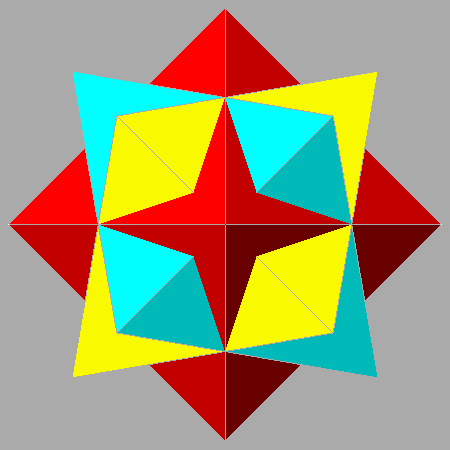Fig.3

In order to get the Escher's solid, it is necessary to substitute a flatter square dipyramid for the octahedron.
Then, in Fig. 4,the animated sequence made of seventeen frames shows a series of compounds of 3 square dipyramids, obtained decreasing progressively the angle between the normal to the dipyramidal faces and the vertical  4-fold axis, from 54.74° = arccos(1/√3), angle between the normal to the {111} faces of the octahedron and the  direction, to the final value of 45° = arccos(1/√2).
Considering a monometric system of Cartesian axes, in correspondence of such 45° angle the Miller's indices relative to the square dipyramid assume the values {11√2}; the association of this dipyramid with two other square dipyramids, obtained by 90° rotations around the  and  directions, reproduces exactly the Escher solid.
The presence of an irrational value among the Miller's indices is due to the fact that we are not dealing with a crystal, which would be subject to the Hauy's law stating that the ratios of the indices are rational numbers.
The faces of the square dipyramid generating the Escher's solid are isosceles triangles, in which the ratio R between each of the two equal sides and the third side corresponds to √3 /2 (as one can infer from geometrical considerations relative to Escher's solid, leading to R2 - (1/2)2 = (1/2)2 + (1/2)2 ; consequently, in the isosceles triangles the two equal angles measure 54.74° = arccos(1/√3).Fig.4

The left column of Fig. 5 shows, in succession, the initial {111} octahedron, the final {11√2} square dipyramid and an animated stereographic projection illustrating the transition between the two single forms; in turn, the right column shows the compound of three octahedra, the Escher's solid and an animated stereographic projection illustrating the transition between the two compounds.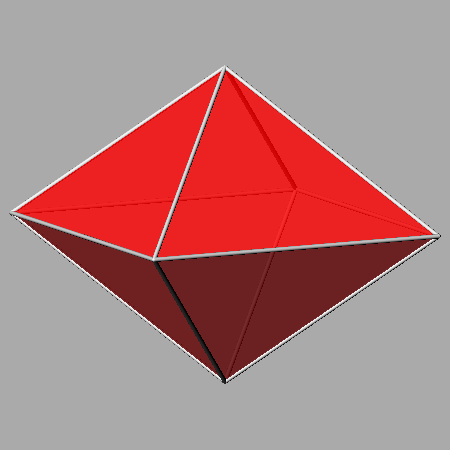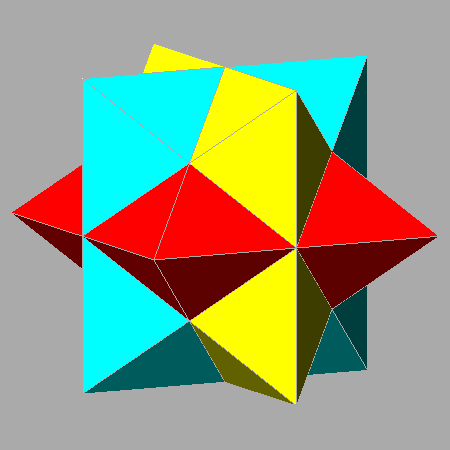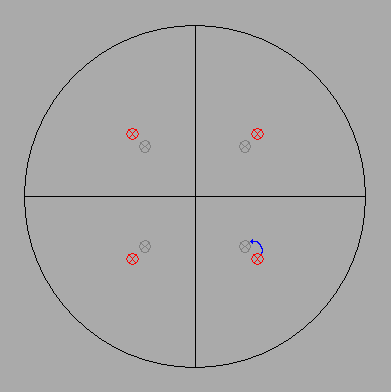Fig.5

It is interesting to note that the skeletal forms of both compounds appear in different versions of Escher's wood engraving Stars (1948) : the compound of three dipyramids, together with other polyhedra, in a preliminary study of the work , whereas the compound of three octahedra is the main subject of the final version .
Fig. 6 shows the remake, along the same angle of view, of both skeletal compounds of three polyhedra drawn by Escher in the successive versions of Stars.

(clicking on the following images, one can visualize the corresponding VRML files)
Fig.6

Substantially, the octahedron can be described as a particular square dipyramid whose faces have the shape of equilateral triangles; because of the presence of four 3-fold axes normal to the faces, in addition to the three 4-fold axes, the six 2-fold axes, the nine mirrors and the inversion center , the relative point group symmetry is 4/m 3 2/m.
The faces of each other square dipyramid are instead isosceles triangles: it implies the absence of the 3-fold axes and therefore the relative symmetry is lower than the symmetry of the octahedron.
When all the generic {hkl} forms, neither parallel nor perpendicular to the  4-fold axis, are square dipyramids, the corresponding point group symmetry is 4/m.

Applying to the {11√2} square dipyramid a 45° clockwise rotation around the vertical  axis, one obtains a congruent form characterized by the more usual {101} Miller's indices: in fact, assuming again a monometric system of Cartesian axes, the (101) face of the dipyramid intersects equal parameters on  and  axes.
The animated views of the square dipyramids involved in the 45° rotation and their stereographic representation are shown in Fig. 7 .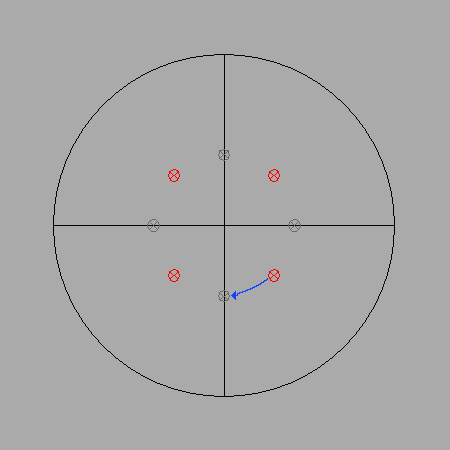Fig.7

Analogously to the procedure previously adopted for the {111} octahedron, a 90° rotation of the red {101} square dipyramid around the  direction leads to a yellow square dipyramid; another 90° rotation of the red dipyramid around the  direction leads to a pale-blue square dipyramid (Fig. 8).
The Escher's solid deriving from the square dipyramids in this new orientation is shown in the fourth image of Fig. 8.

(clicking on the following images, one can visualize the corresponding VRML files)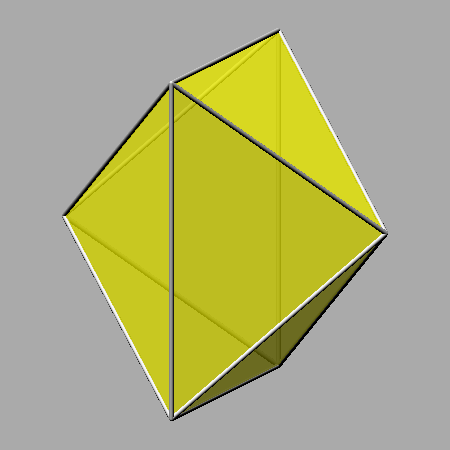Fig.8

In the upper row of Fig. 9 one can see the three compounds made of two square dipyramids, intermediates between the single dipyramid and the Escher's solid.
In each case, four couples of faces belonging to different square dipyramids are partially overlapped: the shared surfaces, differently coloured, have a rhombic shape (with the ratio between the length of the diagonals equal to √2).
As a consequence, the intersection of the three square dipyramids corresponds to a rhomb-dodecahedron (viceversa, the stellation of the rhomb-dodecahedron generates the Escher's solid ). In the lower row of Fig. 9 one can see, on the left, the rhomb-dodecahedron which results from the intersection of the three square dipyramids generating the Escher's solid drawn on the right.
The central image of the lower row represents the stereographic projection, along the  direction, of the rhomb-dodecahedron and also of the faces of the Escher's solid.

(clicking on the following images, one can visualize the corresponding VRML files)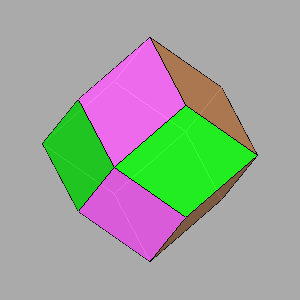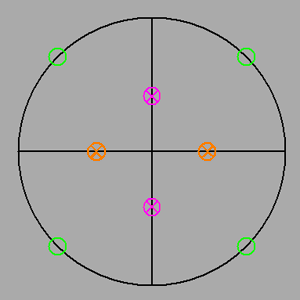Fig.9

Six further views of the Escher's solid are visualized in Fig. 10: the views along the  4-fold axis, the   3-fold axis and the  2-fold axis (in each case with the  direction set vertically) in the upper row, the related clinographic views in the lower row.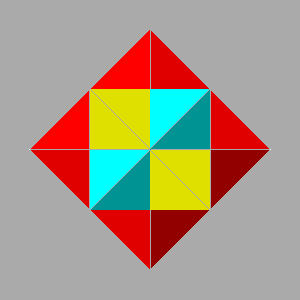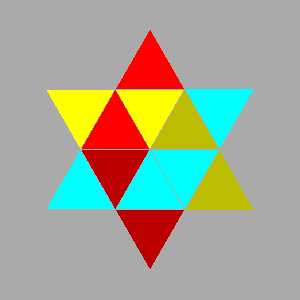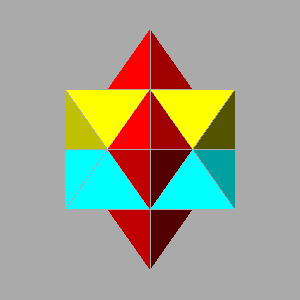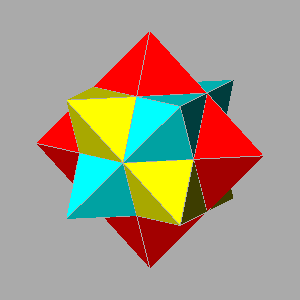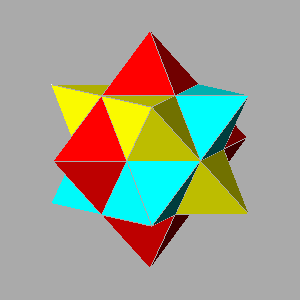Fig.10

Applying also to the {111} octahedron a 45° rotation around the vertical  axis, one obtains a congruent form characterized by the irrational {√201} Miller's indices.
The animated views of the octahedra involved in the 45° rotation and their stereographic representation are shown in Fig. 11.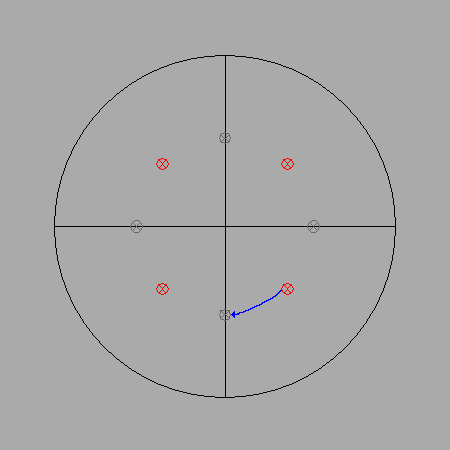Fig.11
The following Fig.12 shows sequentially:
• in the first row: in the center, the red {√201} octahedron and, sideways, the yellow and the pale-blue octahedra obtained by 90° rotations around the  and  directions, respectively
• in the second row: the three compounds of two octahedra (to be compared with the three compounds of two square dipyramids drawn in Fig. 9).
One can note that they are congruent by 90° rotations around the cartesian axes:
• a 90° rotation around  involves the compound made of the red and the yellow octahedra and the compound made of the red and the pale-blue octahedra
• a 90° rotation around  involves the compound made of the red and the pale-blue octahedra and the compound made of the yellow and the pale-blue octahedra
• a 90° rotation around  involves the compound made of the yellow and the pale-blue octahedra and the compound made of the red and the yellow octahedra
• in the third row: the three solids, derived from the intersection of each couple of octahedra, they too congruent by 90° rotations around the cartesian axes; their sixteen faces consist of eight trapezoids (or trapezia) and eight kites (or deltoids).
Their main geometrical features are the following:
• the inner angles of the trapezoids measure 112.21° and 67.79° (ratio between the length of the parallel sides equal to 2)
• the angle between the short sides of the kites measures 98.21°
• the angle between the long sides of the kites measures 78.46°
• the two angles between the sides of the kites having different length measure 91.66°.
• in the fourth row: the relative stereographic projections; as evidenced by the central image, 4/m 2/m 2/m is the maximum point symmetry that can be associated both to a compound of two octahedra and to the solid resulting from their intersection.
The 4-fold axis is associated to the ,  and  direction for the red and pale-blue, yellow and pale-blue, red and yellow couples of octahedra, respectively.
(clicking on the following images, one can visualize the corresponding VRML files)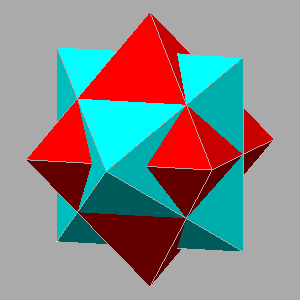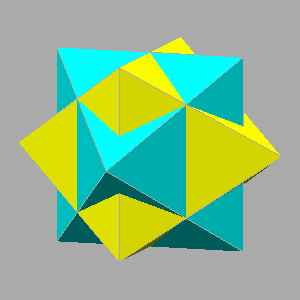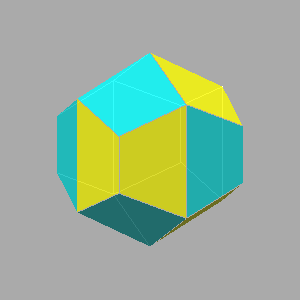Fig.12

The solid derived from the intersection of the three octahedra corresponds to the {√201} tetrakis-cube (or tetrakis-hexahedron), polyhedron with 24 faces that can be derived from a cube in which, by a process of  cumulation  (or augmentation ), a square pyramid grows on each face of the cube. In Fig. 13 one can see, on the left, the tetrakis-cube, on the right the compound of 3 octahedra of which the tetrakis-cube is the intersection, and, in the center, the stereographic projection along , common to both: the relative point symmetry is 4/m 3 2/m, higher than the 4/m 2/m 2/m point symmetry of the compound of two octahedra and of the solid resulting from their intersection.
One can notice that the orientation of all the rotation axes relative to the compound of three octahedra derived from the initial red {√201} octahedron is the usual orientation of the rotation axes in the 4/m 3 2/m cubic point group, relative also to the tetrakis-cube: 4-fold axis along , 3-fold axis along , 2-fold axis along , and the respective equivalent directions.
On the other hand, in case of the association of three octahedra starting from the {111} octahedron, as shown previously in the stereographic projection of Fig. 3, the orientation of the rotation axes looks uncommon: 4-fold axis along , 3-fold axis along [0 √2 1], 2-fold axis along  and the respective equivalent directions.
In practice, the different orientation in the two cases of the axes of symmetry consists in a 45° rotation around the  direction.

(clicking on the image of the tetrakis-cube, one can visualize the corresponding VRML file)
Fig.13

Incidentally, one must avoid to mistake the previous {√201} tetrakis-cube for the Catalan {201} tetrakis-cube, dual of the Archimedean truncated octahedron [11,12], in which the height of the square pyramids augmenting the faces of the cube is lower than in {√201} tetrakis-cube.
In Fig. 14, the Catalan tetrakis-cube is drawn in the upper row, on the left: it corresponds to the intersection of three square dipyramids, whose compound, derived from the red {201} square dipyramid, is shown on the right.
The dual of the Catalan tetrakis-cube is the Archimedean truncated octahedron shown in the lower row, on the left, together with the compound of an octahedron and a cube of which it is the intersection; the ratio between the central distances of the octahedral and cubic faces is equal to √3/2.

(clicking on the following images one can visualize the corresponding VRML files)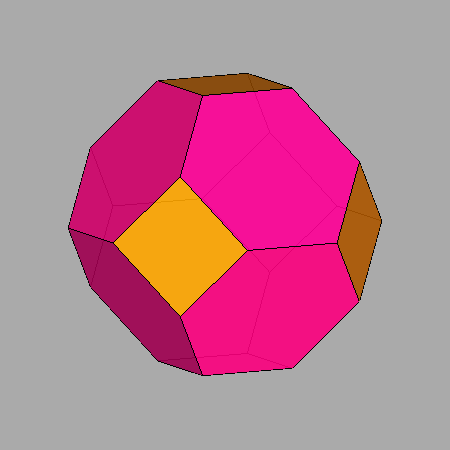Fig.14

Even if, as already pointed out, the (101) face of the square dipyramid and the (111) face of the octahedron make different angles with the vertical  direction, both belong to the zone of the  direction, like so, in case of the 4/m point group, all the square dipyramids {hkh} with 0 < k/h < 1, intermediate between the {101} dipyramid and the {111} octahedron.
The left column of Fig.15 reports a series of six polyhedra from the {101} square dipyramid to the {111} octahedron, passing through the {hkh} dipyramids: {414}, {525}, {212}, {434}; the series on the right includes the corresponding compounds of 3 polyhedra, from the Escher's solid to the compound of three octahedra.{101} square dipyramid and the relative compound of 3 dipyramids corresponding to the Escher's solid{414} square dipyramid and the relative compound of 3 dipyramids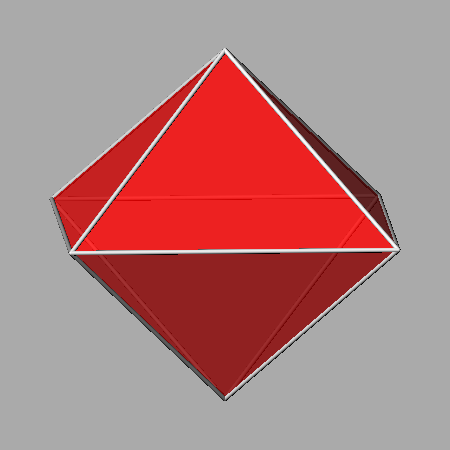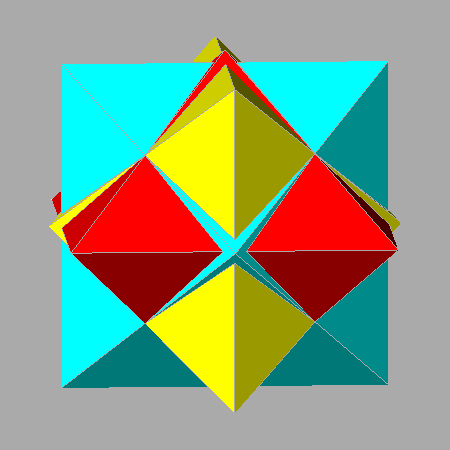{525} square dipyramid and the relative compound of 3 dipyramids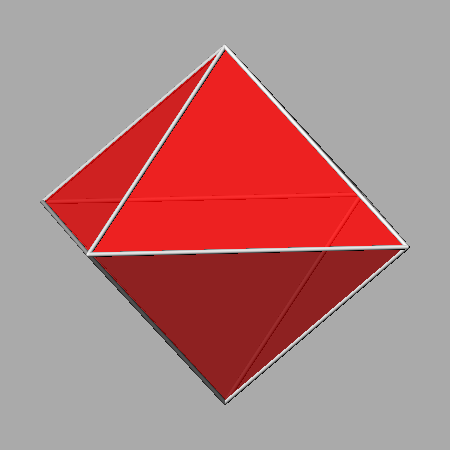{212} square dipyramid and the relative compound of 3 dipyramids{434} square dipyramid and the relative compound of 3 dipyramids{111} octahedron and the relative compound of 3 octahedra
Fig.15

The compounds of three dipyramids derived from {hkh} square dipyramids, shown in Fig.15, are congruent with the compounds of the sequence reported in Fig.4, relative to compounds of three dipyramids derived from {h'h'l'} square dipyramids included between the {111} octahedron and the {11√2} dipyramid, where:  h'/l' = √(k/h)2 +1 /√2 .

It is interesting to remark that if the initial polyhedron is whichever {h0l} square dipyramid, the two other square dipyramids belonging to the corresponding compound of three dipyramids can be alternatively obtained by 120° and 240° rotations of the first dipyramid around the  direction.
This direction correspond to one of the four 3-fold axes (the others are along: , , [1 1 1] ) present in all the compounds of three square dipyramids deriving from whichever {h0l} dipyramid, included the Escher's solid derived from the {101} dipyramid, and in the compound of three octahedra derived from the {√201} octahedron.
Similarly, when the initial polyhedron is the {111} octahedron or whichever {h'h'l'} square dipyramid, included the {11√2} dipyramid generating the Escher's solid, the 120° and 240° rotations are around the [0 √2 1] 3-fold axis (or one of the three other equivalent directions: [0 √2 1], [√2 0 1], [√2 0 1]) characterizing these other compounds of three square dipyramids.
Consequently, one can wonder which [uvw] direction corresponds to a 3-fold axis of each compound of three square dipyramids derived from a square dipyramid {hkh} with 0 < k/h < 1,
Relatively to a series of such compounds of three dipyramids, in the four columns of Table 1 one can find, in sequence:

• the {hkh} indices, with 0 ≤ k/h ≤ 1, relative to the initial dipyramid from which the compound of three dipyramids can be generated by two 120° and 240° rotations around the 3-fold rotation axis reported in the last column
• the [uv0] direction corresponding to one of the three 4-fold rotation axes of the compound of 3 dipyramids
• the [u'v'0] direction corresponding to one of the six 2-fold rotation axes of the compound of 3 dipyramids
• the [u'v'w'] direction corresponding to one of the four 3-fold rotation axes of the compound of 3 dipyramids
 Rotation axes of the compounds of 3 dipyramids deriving from the {hkh} square dipyramids initial square dipyramid {hkh} 4-fold axis [uv0] 2-fold axis [u'v'0] 3-fold axis [u'v'w'] {101}    {717}   [6 8 5√2] {616}   [5 7 √37] {515}   [4 6 √26] {414}   [3 5 √17] {313}   [2 4 √10] {212}   [1 3 √5] {323}   [1 5 √13] {434}    {545}   [1 9 √41] {656}  [1 11 0] [1 11 √61] {111}   [0 √2 1]
Table 1

The ratio u/v between the first two indices of the direction [uv0] coinciding with a 4-fold axis of each compound of 3 dipyramids corresponds to the ratio h/k between the {hkl} indices of the dipyramid which generates the compound of three dipyramids: u/v = h/k

In particular, for {hkh} dipyramids it implies:
u = h  and  v = k

The [u'v'0] 2-fold axis of a compound of three dipyramids makes an angle of 45° with the [uv0] 4-fold axis of the same compound.
It follows that the ratio u'/v' between the first two indices of a direction [u'v'0] corresponds to the ratio between the difference and the sum of the couples of indices u,v and h,k:

u'/v' = (u-v)/(u+v) = (h-k)/(h+k)

The [u'v'w'] 3-fold axis of whichever compound makes an angle of 54.74° = arccos (1/√3) with the  vertical direction (always coinciding with a further 4-fold axis in all the compounds) and a complementary angle of 35.26° = arctan (1/√2) with the horizontal [u'v'0] 2-fold axis of the same compound of 3 dipyramids. From:

cos 54.74° = 1/√3 = w'/√u'2 + v'2 + w'2    or   tan 35.26° = 1/√2 = w'/√u'2 + v'2
one can infer that:
w' = √(u'2 + v'2)/2
It is easy to verify that:
w'/v' = √h2 + k2/(h+k)

and therefore, taking into account the ratio u'/v' previously calculated, the values of the [u'v'w'] indices relative to one of the four 3-fold axes of a compound of 3 dipyramids derived from a {hkh} dipyramid are the following:

u' = h-k,    v' = h+k,    w' = √h2 + k2

The directions of the three other 3-fold axes of the compound of 3 dipyramids can be obtained by sequential 90° rotations of the first 3-fold axis around the  vertical 4-fold axis; then the directions of the four 3-fold axes are:

[h-k  h+k √h2 + k2],  [-(h+k)  h-k √h2 + k2],  [-(h-k) -(h+k) √h2 + k2],  [h+k  -(h-k) √h2 + k2]

As already pointed out for the {h0l} and {hhl} square dipyramids, all the compounds of three dipyramids which derive from {hkl} square dipyramids, whose indices are characterized by the same h/k ratio, share all the directions of the rotation axes; for istance, in the compounds of three dipyramids deriving from initial {43l} square dipyramids, that is with h/k = 4/3 and variable values of l, a 4-fold axis is directed along , a 2-fold axis along  and a 3-fold axis along .
In Table 2 one can see the complete list of the rotation axes relative to the compounds of three dipyramids derived from {hkl} square dipyramids in which h/k = 4/3.

 Complete list of the rotation axes present in the compounds of 3 dipyramids deriving from an initial {hkl} square dipyramid in which h/k = 4/3 three 4-fold axis six 2-fold axis four 3-fold axis  ,  ,   ,   ,  , [1 7 5] ,   ,  , [4 3 5] , 
Table 2

Since each of the last four 2-fold axes in the list makes an angle of 45° with the couples of 4-fold axes  and  or  and , the indices of the corresponding direction can be identified unambiguously.

The left image in the upper row of Fig.16 visualizes an animated sequence which includes a series of compounds of three square dipyramids, each deriving from a {hkl} square dipyramid characterized by the ratios:  h/k = 4/3  and  1/12l/k ≤ 16 ; it was necessary to rescale progressively the frames of the sequence when l/k ≤ 1 because the dipyramids were becoming always more sharpened.
The other images in the figure consist in three frames of the sequence which are particularly noteworthy: the right image of the upper row shows the compound generated from the {434} square dipyramid, belonging to the zone of  direction and intermediate between the compound generated from the {8 6 5√2} octahedron (left image of the lower row) and the Escher's solid generated from the {435} dipyramid (right image of the lower row).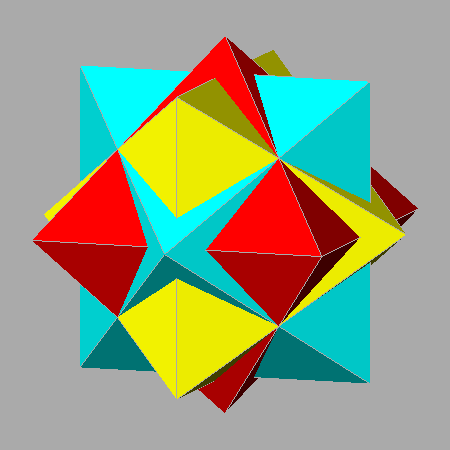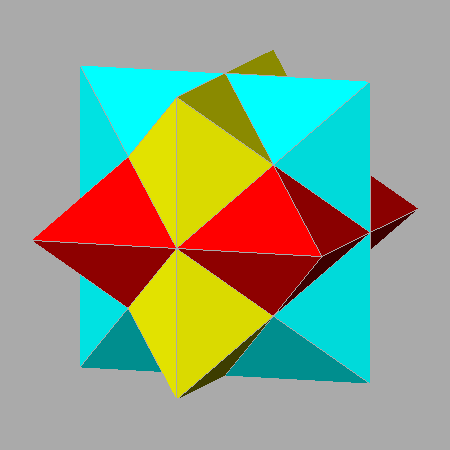Fig.16

More in general, concerning the series of {hkh} square dipyramids with 0 < k/h < 1 reported in the central column of Table 3for each value of the ratio k/h one can find the values of the third Miller's index l relative to the octahedron or to the square dipyramid belonging to the Escher's solid.
Since the normal to their faces makes angles of 54.74° and 45°, respectively, with the vertical  4-fold axis, from:

cos 54.74° = 1/√3 = 1/(h2/ l2)+ (k2/l2) + 1    and     cos 45° = 1/√2 = 1/√(h2/ l2)+ (k2/l2) + 1

one obtains, in the two cases,  l' = √h2 + k2)/2 for the third index of each rotated octahedron and  l" = √h2 + k2 for the third index of each rotated dipyramid generating an Escher's solid.

 {h k √h2 + k2)/2} initial octahedra belonging to each compound of 3 octahedra {hkh} polyhedra in zone with  having {hkh} indices such as: 0 ≤ k/h ≤ 1 {h k √h2 + k2} initial square dipyramids belonging to each compound of 3 dipyramids {√2 0 1} {101} {101} {715} {717} {7 1 5√2} {12 2 √74} {616} {6 1 √37} {5 1√13} {515} {5 1 √26} {8 2 √34} {414} {4 1 √17} {3 1 √5} {313} {3 1 √10} {10 4 √58} {525} {5 2 √29} {4 2 √10} {212} {2 1 √5} {6 4 √26} {323} {3 2 √13} {8 6 5√2} {434} {435} {10 8 √82} {545} {5 4 √41} {12 10 √122} {656} {6 5 √61} {111} {111} {1 1 √2}
Table 3

In Fig.17, the left and the right images consist in the animated sequences of frames describing the 45° rotation underwent by the compound of three octahedra and the Escher's solid, respectively.
In the central image, the first frame of the sequence concerns the compound of 3 dipyramids (derived from the {101} square dipyramid) corresponding to an Escher's solid, that coincide with the first frame of the third sequence, whereas the last central frame concerns the compound of 3 octahedra (derived from the {111} octahedron) that coincides with the the last frame of the first sequence.Fig.17